# 1. Evaluate (by SHOWING YOUR WORK! You CANNOT use DE L'HOPITAL's RULE!!) the following limits. If...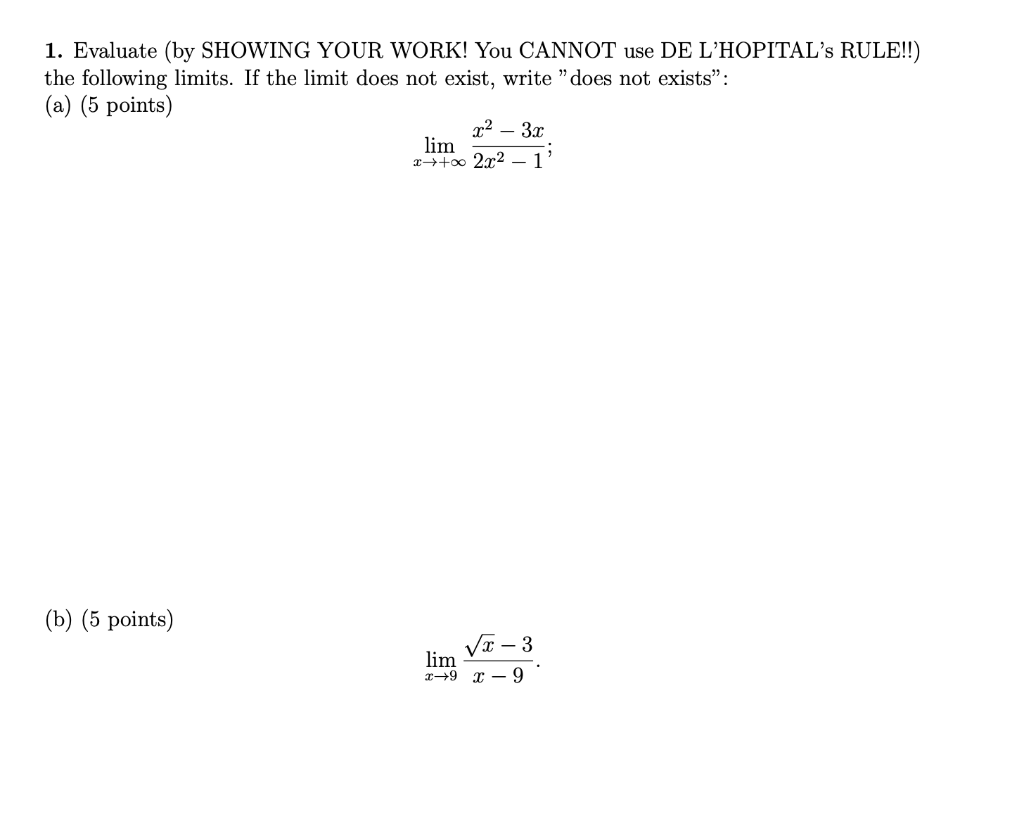1. Evaluate (by SHOWING YOUR WORK! You CANNOT use DE L'HOPITAL's RULE!!) the following limits. If the limit does not exist, write "does not exists”: (a) (5 points) x2 - 32 lim ++ 2x2 - 1' (b) (5 points) - 3 lim 169 - 9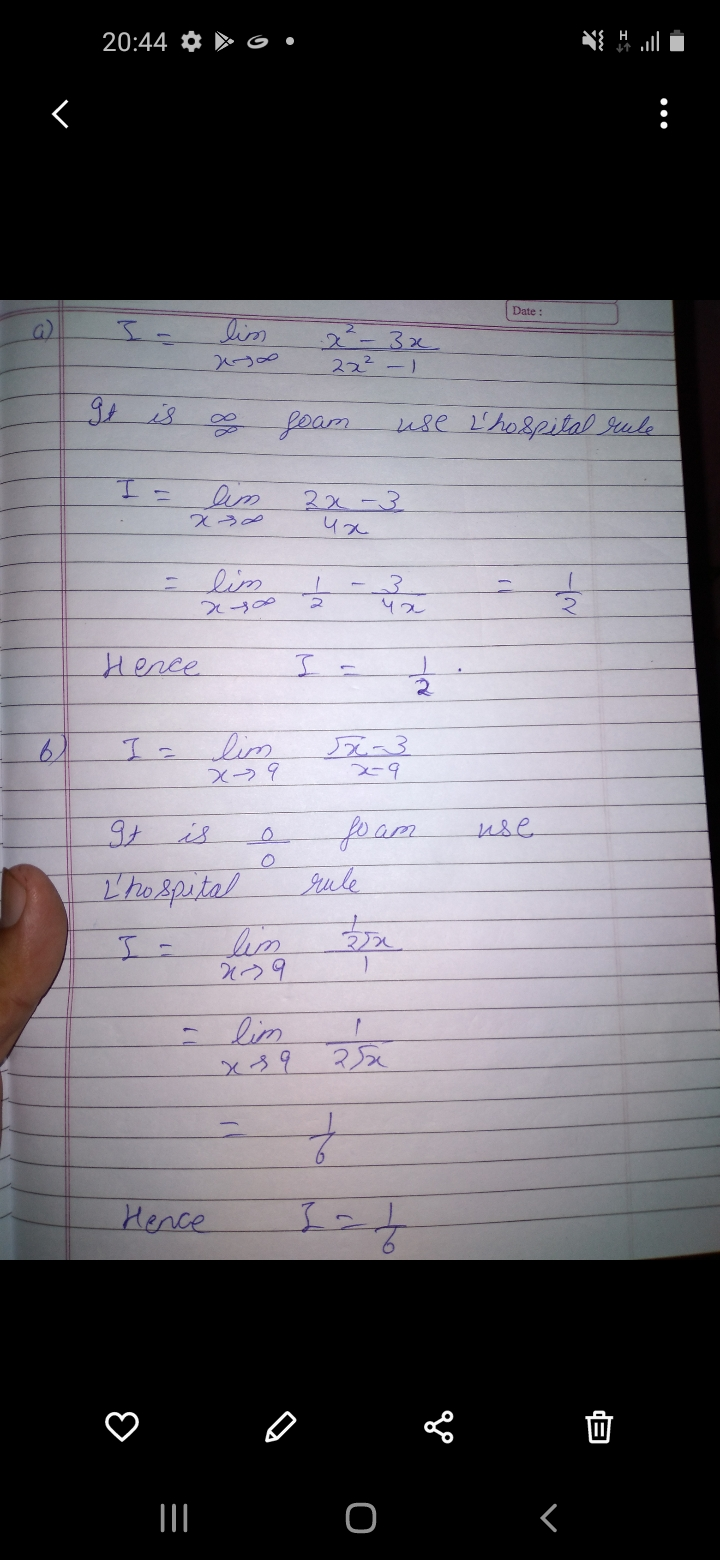please like

#### Earn Coin

Coins can be redeemed for fabulous gifts.

Similar Homework Help Questions
• ### 1. Evaluate (by SHOWING YOUR WORK! You CANNOT use DE L'HOPITAL's RULE!!) the following limits. If...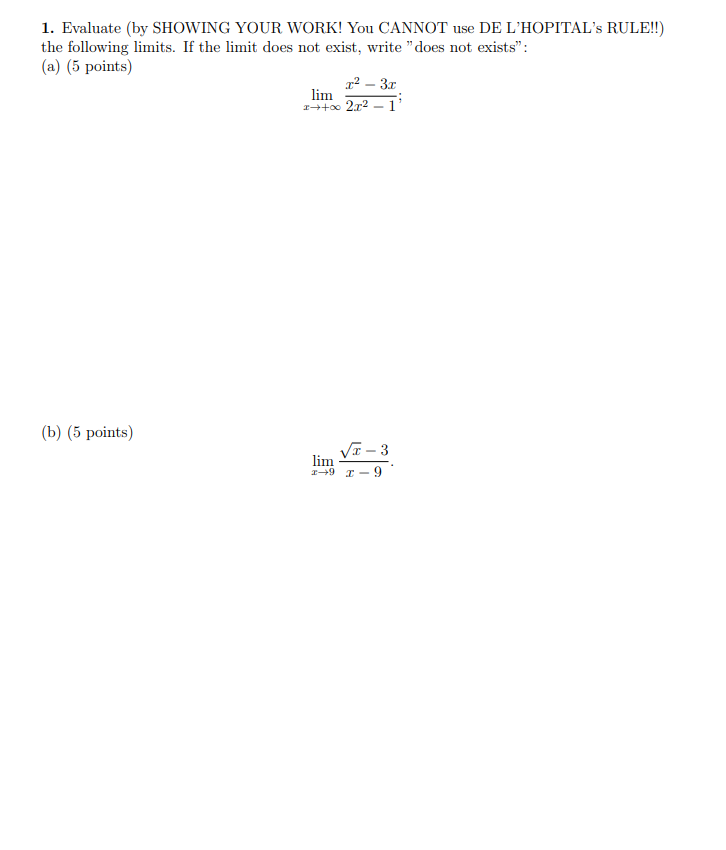1. Evaluate (by SHOWING YOUR WORK! You CANNOT use DE L'HOPITAL's RULE!!) the following limits. If the limit does not exist, write "does not exists": (a) (5 points) 2 - 31 lim 1++ 2.12 (b) (5 points) Vr-3 lim +91-9

• ### 2. Evaluate (by SHOWING YOUR WORK!) the following limits. If the limit does not exist, write...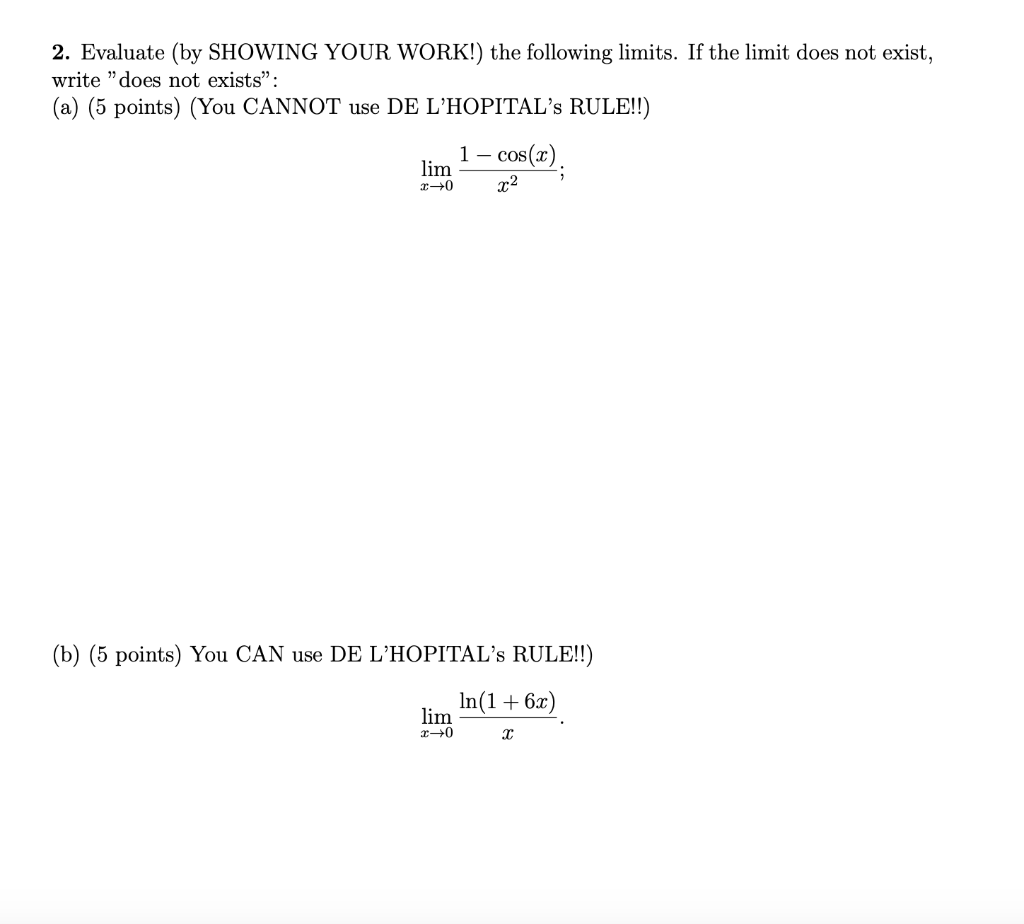2. Evaluate (by SHOWING YOUR WORK!) the following limits. If the limit does not exist, write "does not exists": (a) (5 points) (You CANNOT use DE L'HOPITAL's RULE!!) 1 – cos(x). lim 20 x2 (b) (5 points) You CAN use DE L'HOPITAL's RULE!!) In(1+6x) lim 10 2

• ### 2. Evaluate (by SHOWING YOUR WORK!) the following limits. If the limit does not exist, write...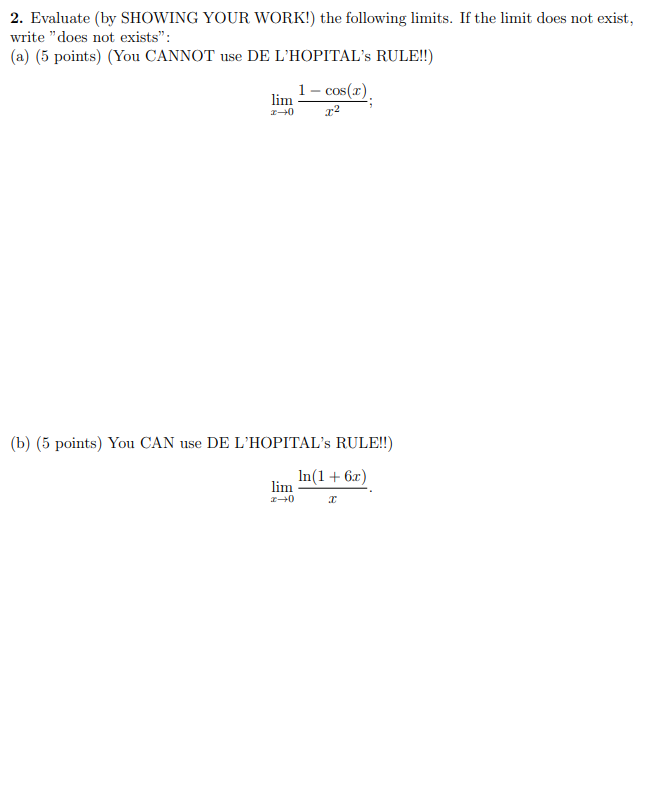2. Evaluate (by SHOWING YOUR WORK!) the following limits. If the limit does not exist, write "does not exists": (a) (5 points) (You CANNOT use DE L'HOPITAL'S RULE!!) lim 1 - cos(x). 1-0 .22 (b) (5 points) You CAN use DE L'HOPITAL'S RULE!!) In(1 +6x) lim 2-0 C

• ### Evaluate the following limits. If you use L'Hopital's Rule, indicate on your paper that you have...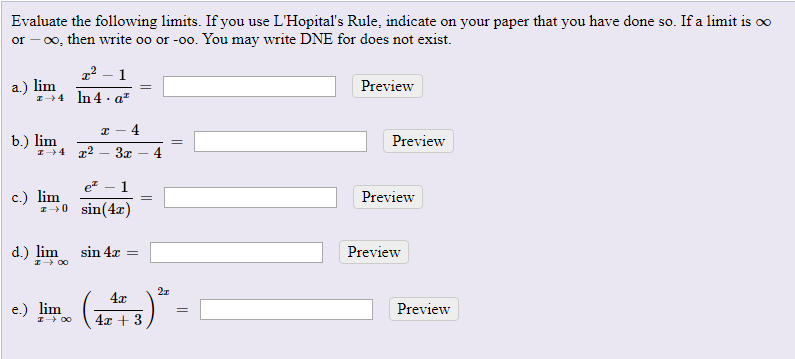Evaluate the following limits. If you use L'Hopital's Rule, indicate on your paper that you have done so. If a limit is oo or - 0, then write oo or -oo. You may write DNE for does not exist. x² – 1 a.) lim Preview 7+1 In 4.q7 = - b.) lim 1+ I-4 2 – 3. - 4 Preview et -1 c.) lim 1+0 - sin(4x) Preview d.) limsin 4x = Preview Preview

• ### explain clearly please Problem 3 (6 points each). Evaluate the following limits algebraically, showing all work....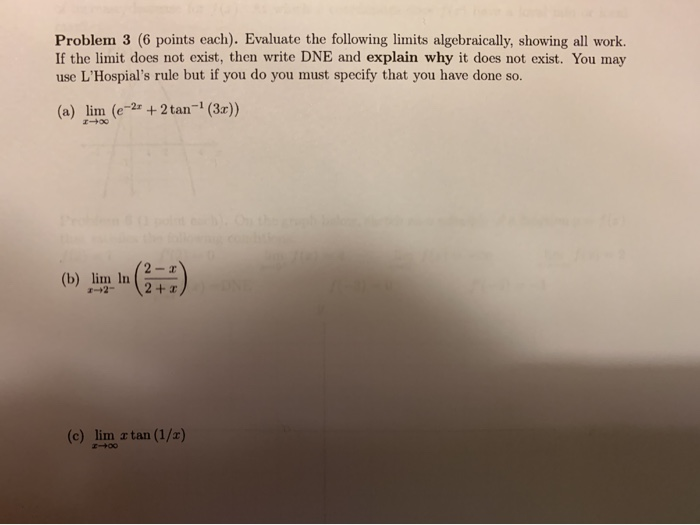explain clearly please Problem 3 (6 points each). Evaluate the following limits algebraically, showing all work. If the limit does not exist, then write DNE and explain why it does not exist. You may use L'Hospial's rule but if you do you must specify that you have done so. (a) lim (e-2x + 2 tan-? (3.)) (5) limun (2+1) (c) lim a tan (1/1)

• ### 1. Find the following limits (You are not allowed to use L'Hopital's Rule) : x2 +...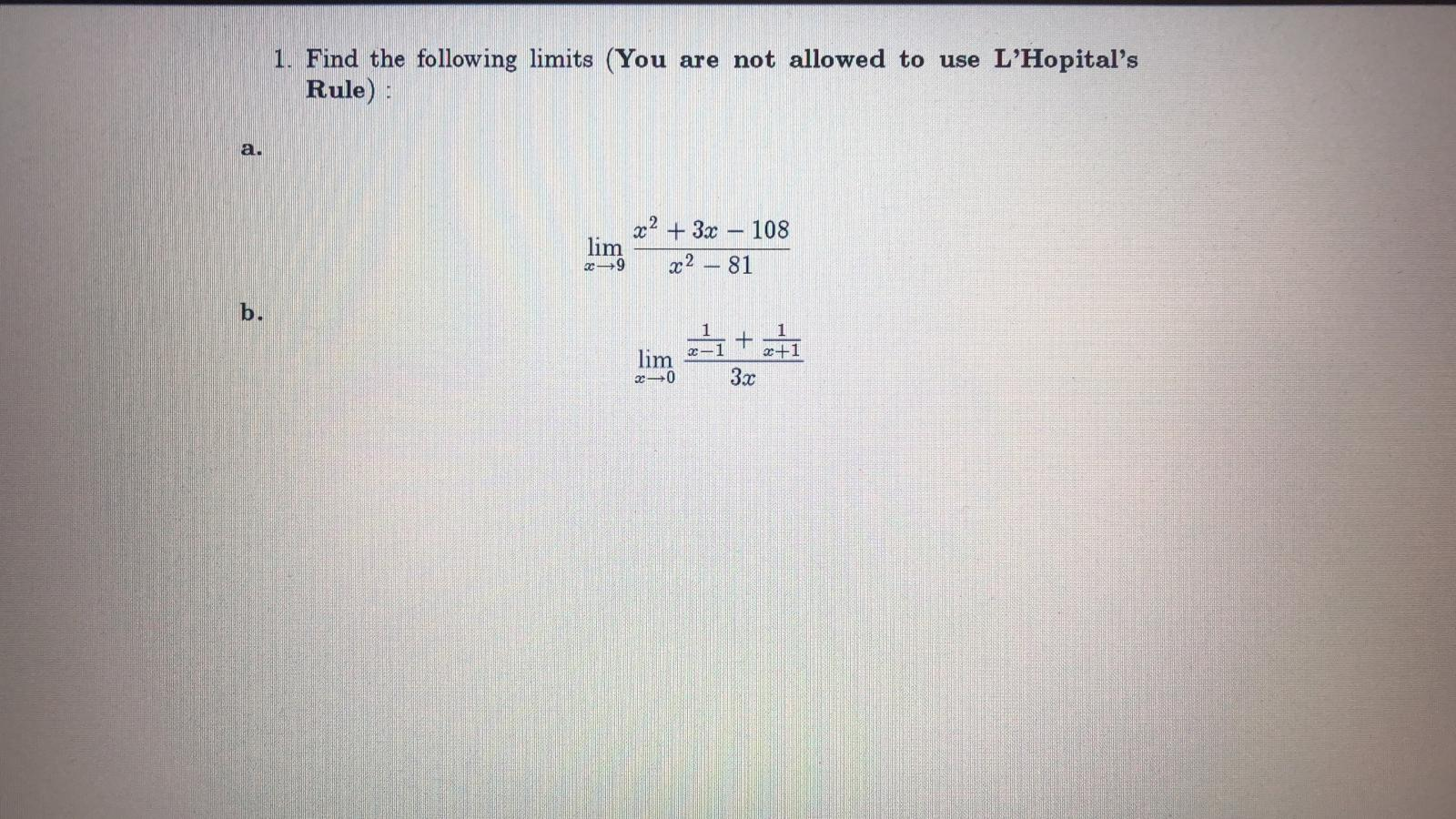1. Find the following limits (You are not allowed to use L'Hopital's Rule) : x2 + 3x – 108 din 22 – 81 –0 32

• ### 6. Use l'Hopital's rule to evaluate the following limits 1+cos (Te sin(z) (a) lim z-+0 log...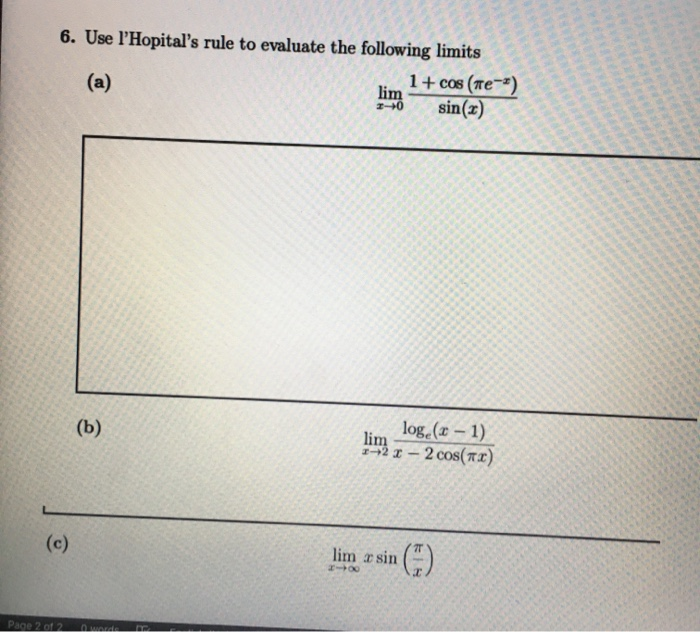6. Use l'Hopital's rule to evaluate the following limits 1+cos (Te sin(z) (a) lim z-+0 log (-1) (b) lim 92I-2 cos(TI) (c) lim r sin Page 2 of 2 0 words

• ### Use series representation(s) to evaluate the following limit (You may not use L'Hopital's rule). . X...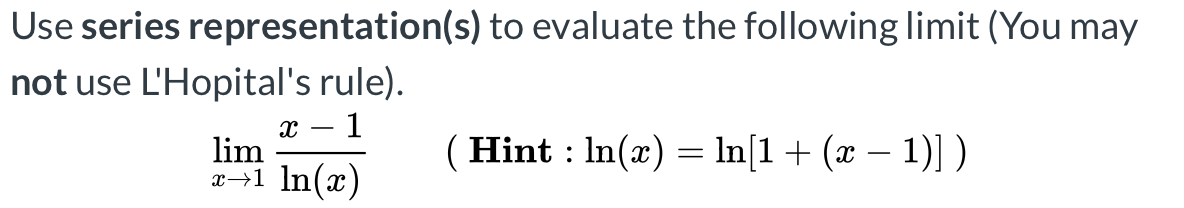Use series representation(s) to evaluate the following limit (You may not use L'Hopital's rule). . X – 1 (Hint : ln(x) = ln(1 + (x – 1)]). x+1 ln(x) lim

• ### (a) For each of the following, determine if L'Hopital's Rule applies. lim * x2+2x–15 cos(x-3)-1 (No...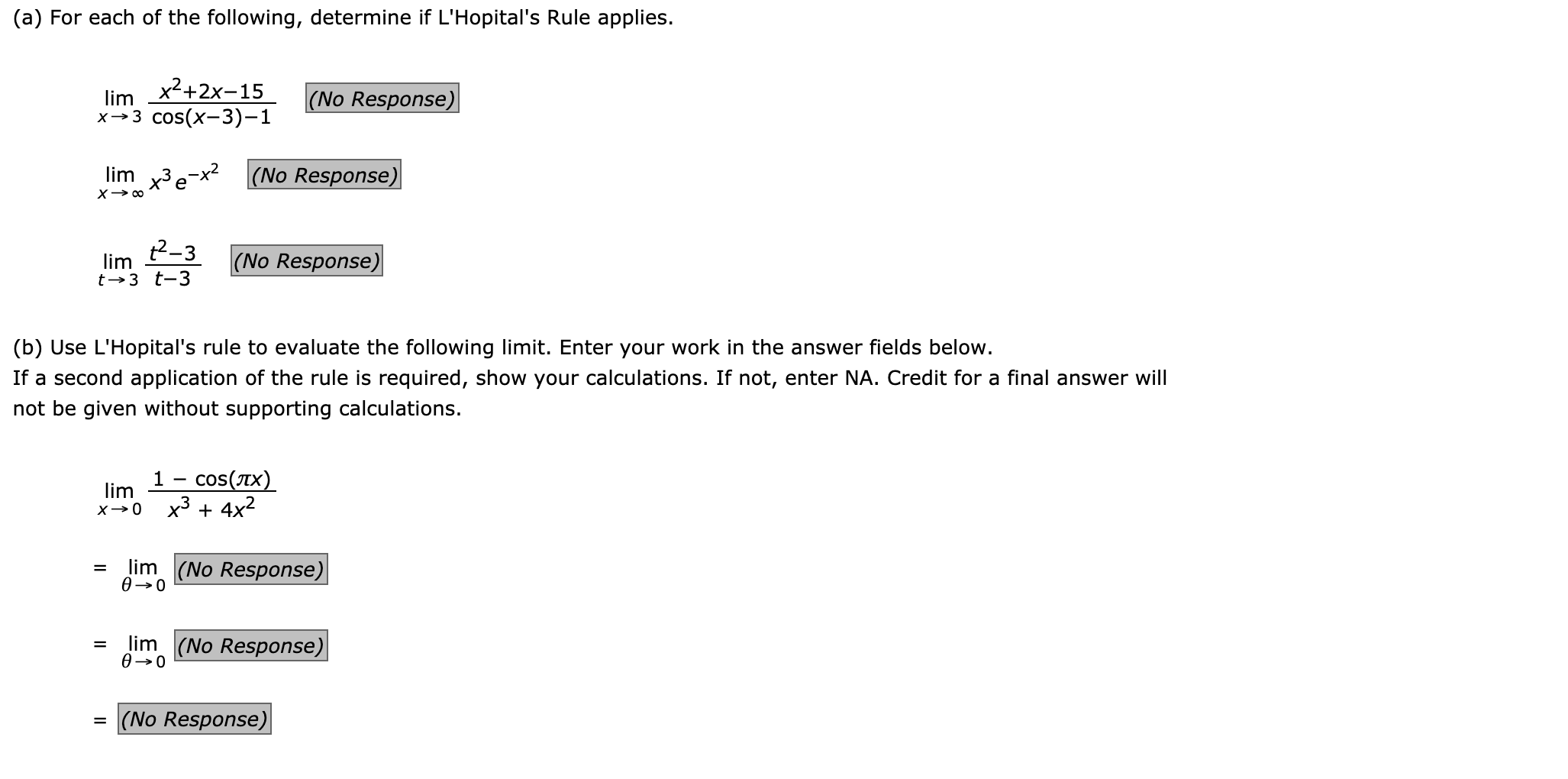(a) For each of the following, determine if L'Hopital's Rule applies. lim * x2+2x–15 cos(x-3)-1 (No Response) lim x3 e-x2 (No Response) x → ^ lim lim t3 ? t-3 3 (No Response) Vo Resp (b) Use L'Hopital's rule to evaluate the following limit. Enter your work in the answer fields below. If a second application of the rule is required, show your calculations. If not, enter NA. Credit for a final answer will not be given without supporting calculations....

• ### Determine if the following limits have an indeterminant form, state it, and use L'Hopital's rule to...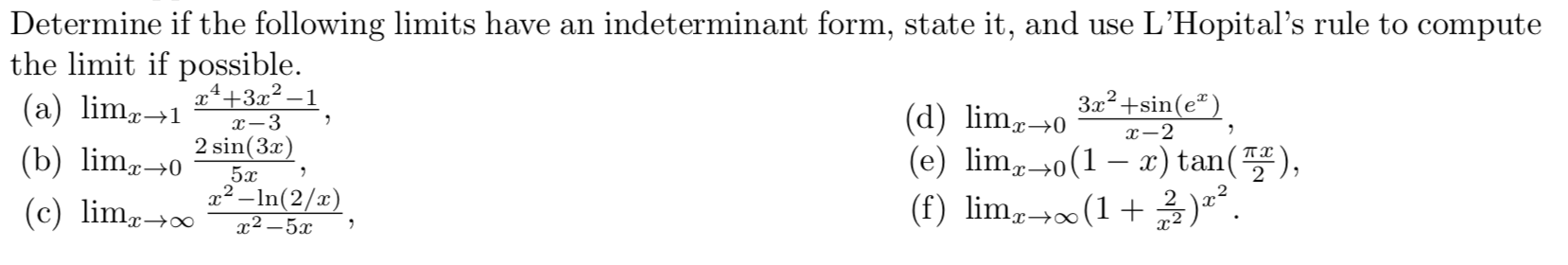Determine if the following limits have an indeterminant form, state it, and use L'Hopital's rule to compute the limit if possible. (a) limz-x++3x2–1 1 -3 (d) lim240 32° +sin(e"), (b) lime- 2 sin(3x) (e) lime-o(1 – x) tan(7), x2 – In(2/x) (f) limo+(1+2).. X-2 5.0 (c) lime+o022 –52